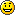# An ODE, Orthogonal Functions, and the Chebyshev Polynomials

Orthogonal functions are very useful and rather easy to use.  The most famous ones are sin(n x) and cos(n x) on the interval [$-\pi$, $\pi$].  It is extremely easy to do linear regression with orthogonal functions.

Linear regression involves approximating a known function $f(x)$ as the weighted sum of other functions.  This shows up all the time in various disguises in machine learning:

• When using AdaBoosting we perform a weighted sum of weak classifiers.
• Support vector machines find a linear combination of the features that separates the data into two classes.
• Each node in a neural net typically computes a weighted sum of the nodes in the prior layer.

For orthogonal functions, computing the linear regression is simple.  Suppose that the features are $f_1, f_2, \ldots, f_n$ and the functions $f_i$ are orthonormal, then the best approximation of the function $g(x)$ is the same as the solution to the linear regression

$$g(x) \approx \sum_{i=1}^n \alpha_i f_i(x)$$

where $\alpha_i$ is the “inner product” of $f_i$ and $g$ denoted

$\alpha_i= \langle\;g, f_i\rangle = \int g(x) f(x) w(x) dx.$  (Two function are orthogonal if their inner product (or dot product) is zero.  The set of orthogonal functions $\{f_i\}$ is called orthonormal if $<f_i, f_i>=1$ for all $i$.)  The function $w(x)$ is a positive weight function which is typically just $w(x)=1$.

For example, if we try to approximate the function $g(x)=x^2$ on the interval [-1,1] by combining the features $f_1(x) = 1/\sqrt(2)$ and $f_2(x) = x \sqrt{3 \over 2}$, then

$$g(x) \approx \sum_{i=1}^n \alpha_i f_i(x)$$

where

$$\alpha_1 = \langle g, f_1\rangle = \int_{-1}^1 g(x) f_1(x) dx = \int_{-1}^1 x^2 1/\sqrt2 dx = \sqrt{2}/3,$$

$$\alpha_2 = \langle g, f_2\rangle = \int_{-1}^1 g(x) f_2(x) dx= \int_{-1}^1 x^2 \sqrt{3/2}\; x dx =0,$$

and the best approximation is then $\sum_{i=1}^n \alpha_i f_i(x) = \alpha_1 f_1(x) = 1/3$.

The other day I needed to do a linear regression and I wanted to use orthogonal functions, so I started by using the trigonometric functions to approximate my function $g(x)$.  Using trig functions to approximate another function on a closed interval like [-1,1] is the same as computing the Fourier Series.  The resulting approximations were not good enough at x=-1 and x=1, so I wanted to change the weight function $w(x)$.  Now assume that $u_1(x), u_2(x), \ldots, u_n(x)$ are orthonormal with the weight function w(x) on [-1,1].  Then

$$\int_{-1}^1 u_i(x) u_j(x) w(x) dx = \delta_{i,j}$$

where $\delta_{i,j}$ is one if $i=j$ and zero otherwise.  If we make a change of variable $x=y(t)$, then

$$\int_{y^{-1}(-1)}^{y^{-1}(1)} u_i(y(t)) u_j(y(t)) w(y(t)) y'(t) dx = \delta_{i,j}.$$

It would be nice if

$$(1)\quad w(y(t)) y'(t)=1,$$

because then I could just set $u_i(y(t))$ equal to a trig function and the resulting $u_i(x)$ would be orthonormal with respect to the weight function $w(x)$. Condition (1) on $y$ is really an ordinary differential equation

$$y’ = {1\over{w(y)}}.$$

In order to get a better fit at $x=\pm 1$, I tried two weight functions $w(x) = {1\over{(1-x)(1+x)}}$ and $w(x) = {1\over{\sqrt{(1-x)(1+x)}}}$.  For the former, the differential equation has solutions that look similar to the hyperbolic tangent function. The latter $w(x)$ yields the nice solution

$$y(t) = \sin( c + t).$$

So now if I choose $c=\pi/2$, then $y(t) = \cos(t)$, $y^{-1}(-1) = \pi$, $y^{-1}(1)=0$, so I need functions that are orthonormal on the interval $[-\pi, 0]$.  The functions $\cos(n x)$ are orthogonal on that interval, but I need to divide by $\sqrt{\pi/2}$ to make them orthornormal.  So, I set $u_n(y(t)) = \sqrt{2/\pi}\cos(n\;t)$ and now

$$\int_{-1}^1 {{u_i(x) u_j(x)}\over {\sqrt{(1-x)(1+x)}}} dx = \delta_{i,j}.$$

The $u_n(x)$ functions are orthonormal with respect to the weight $w(x)$ which is exactly what I wanted.

Solving for $u(x)$ yields

$$u_n(x) = \sqrt{2/\pi} \cos( n \;\arccos(x)),$$

$$u_0(x) = \sqrt{2/\pi}, u_1(x) = \sqrt{2/\pi} x,$$

$$u_2(x) = \sqrt{2/\pi}(2x^2-1), u_3(x) = \sqrt{2/\pi}(4x^3-3x),\ldots.$$

If you happen to be an approximation theorist, you would probably notice that these are the Chebyshev polynomials multiplied by $\sqrt{2/\pi}$.   I was rather surprised when I stumbled across them, but when I showed this to my friend Ludmil, he assured me that this accidental derivation was obvious.  (But still fun)

1.This post reminds me of a friend who used to chastise me when I used “orthogonal” when I meant “independent.” For sin x and cos x are orthogonal but they are clearly not independent.

2.Hum..
seems that Mr Tchebychev followed the same thinking path..

1.I wonder if there is some way to know ahead of time or intuit that the result is going to be polynomial.

I guess for any weight function, it’s possible to get orthogonal polynomials, but the Chebyshev polynomials are so nice—integer coefficients and all.